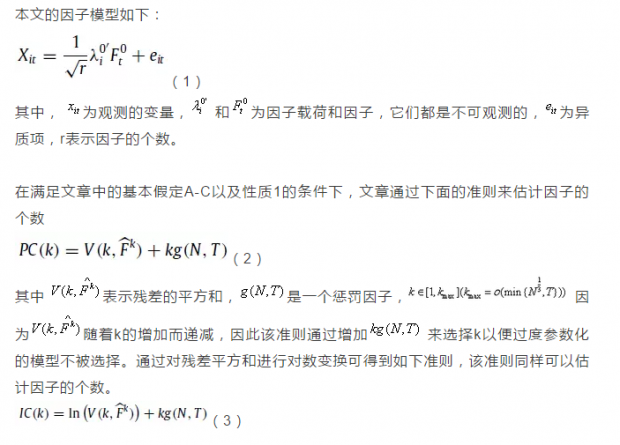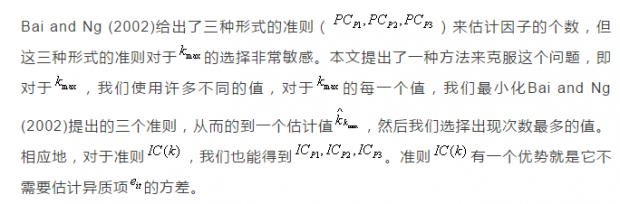## 当因子的个数随样本增加时确定因子的个数

1 引言

2. 方法3. 蒙特卡洛模拟4. 结论

Correctly specifying the number of factors (r) is a fundamental issue for the application of factor models. In this paper we develop an econometric method to estimate the number of factors in factor models of large dimensions where the number of factors is allowed to increase as the two dimensions, cross-section size (N) and time period (T ) increase. Using similar information criteria as proposed by Bai and Ng (2002), we show that the number of factors can be consistently estimated using the criteria. We propose a new procedure that avoids over estimating the number of factors while allowing for one to search for possible number of factors over a wide range of positive integers so that it also avoids underestimation of the number of factors. We conduct Monte-Carlo simulation to investigate the finite sample properties of the proposed approach.